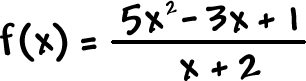What about this one?

 Find the horizontal asymptote of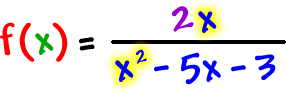Look atSo the horizontal asymptote is the line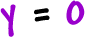(which is the x-axis)

 This one falls under part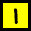on our list.

YOUR TURN:

 Find the horizontal asymptote of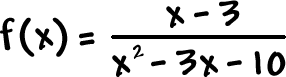OK, so what about this one?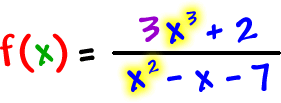If we look at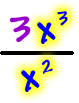, we find that the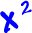WILL divide in... But, there's going to be some x stuff left over to deal with.  This is when you need to start in with some long division...  and we get to ignore the remainder!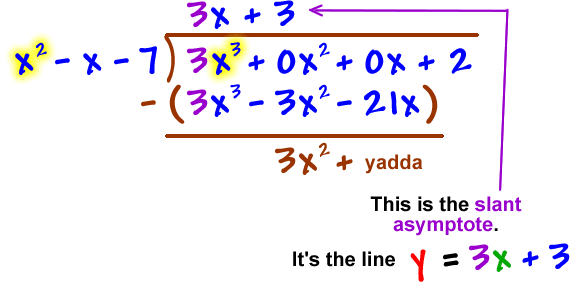You can stop here since the rest will be remainder stuff.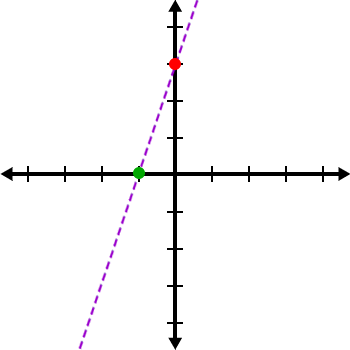TRY IT:

 Find the slant asymptote of